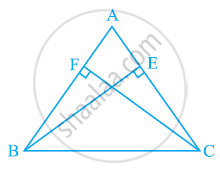Share

# ABC is an isosceles triangle in which altitudes BE and CF are drawn to equal sides AC and AB respectively (see the given figure). Show that these altitudes are equal. - CBSE Class 9 - Mathematics

ConceptProperties of a Triangle

#### Question

ABC is an isosceles triangle in which altitudes BE and CF are drawn to equal sides AC and AB respectively (see the given figure). Show that these altitudes are equal.#### Solution

In ΔAEB and ΔAFC,

∠AEB and ∠AFC (Each 90º)

∠A = ∠A (Common angle)

AB = AC (Given)

∴ ΔAEB ≅ ΔAFC (By AAS congruence rule)

⇒ BE = CF (By CPCT)

Is there an error in this question or solution?

#### APPEARS IN

NCERT Solution for Mathematics Class 9 (2018 to Current)
Chapter 7: Triangles
Ex. 7.20 | Q: 3 | Page no. 124

#### Video TutorialsVIEW ALL 

Solution ABC is an isosceles triangle in which altitudes BE and CF are drawn to equal sides AC and AB respectively (see the given figure). Show that these altitudes are equal. Concept: Properties of a Triangle.
S